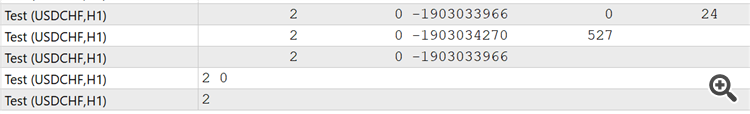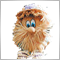# Array problem1480

Hi experts,

I am confused about these code. I have tried to create array in struct.Those initial value should be "0" in all array.

but why not?How does it come from?

```struct line
{
int index[];
};

int count1=0,count2=0;
line test[];

void OnTick()
{

for(int q=0;q<5;q++)
{
ArrayResize(test,count1+1);
count2=0;
for(int w=q;w<5;w++ )
{
ArrayResize(test[count1].index,count2+1);
count2++;
}

count1++;
}

ArrayPrint(test.index);
ArrayPrint(test.index);
ArrayPrint(test.index);
ArrayPrint(test.index);
ArrayPrint(test.index);

}```in my opinion, it should be

0 0

0 0 0

0 0 0 0

0 0 0 0 0

thank you27845

Kittamet Sirinyamas: Those initial value should be "0" in all array.

If you want it initialized, you must do it.1720

you must use ArrayInitialize(test[count1].index,0)1480

Hi guys,
As I know, When declare an array. Any value will initiate at zero.
But this case, there are array with structure.

Why do those values start at different value?27845

Kittamet Sirinyamas: As I know, When declare an array. Any value will initiate at zero.

Wrong. You have been told twice, now three times.

Global and static variables work exactly the same way in MT4/MT5/C/C++.
1. They are initialized once on program load.
2. They don't update unless you assign to them.
3. In C/C++ you can only initialize them with constants, and they default to zero. In MTx you should only initialize them with constants. There is no default in MT5 (or MT4 with strict which you should always use.)

MT4/MT5 actually compiles with non-constants, but the order that they are initialized is unspecified and don't try to use any price or server related functions in OnInit (or on load,) as there may be no connection/chart yet:

1. Terminal starts.
2. Indicators/EAs are loaded. Static and globally declared variables are initialized. (Do not depend on a specific order.)
3. OnInit is called.
4. For indicators OnCalculate is called with any existing history.
5. Human may have to enter password, connection to server begins.
6. New history is received, OnCalculate called again.
7. New tick is received, OnCalculate/OnTick is called. Now TickValue, TimeCurrent, account information and prices are valid.
4. Unlike indicators, EAs are not reloaded on chart change so you must reinitialize them, if necessary.
external static variable - Inflation - MQL4 programming forum1480

I just have got a new problem. hope you guys solve this.

suppose I have this

```struct x
{
int y[];
int z;
};```

then I declare struct as array

`x test[];`

If I want to create some function calling test[]

like this

```void func (.... &test[])
{

}```

this is my question

1.What is prefix or type of &test[]? What type should I fill in this(....)?

2.how to access the member of arraystruct (there are 2 y[] and z) in the function when I pass array with struct ?

thank you againModerator
306178

Kittamet Sirinyamas :

I just have got a new problem. hope you guys solve this.

suppose I have this

then I declare struct as array

If I want to create some function calling test[]

like this

this is my question

1.What is prefix or type of &test[]? What type should I fill in this(....)?

2.how to access the member of arraystruct (there are 2 y[] and z) in the function when I pass array with struct ?

thank you again

I would suggest this option:

```//+------------------------------------------------------------------+
//|                                                      Test_en.mq5 |
//+------------------------------------------------------------------+
struct Sx
{
int               y;
int               z;
//--- Constructor
Sx()
{
ArrayInitialize(y,1);
z=2;
}
//--- Destructor
~Sx()
{
}
};
//+------------------------------------------------------------------+
//| Script program start function                                    |
//+------------------------------------------------------------------+
void OnStart()
{
Sx test[];
ArrayResize(test,3);
//---
func(test,2);
}
//+------------------------------------------------------------------+
//|                                                                  |
//+------------------------------------------------------------------+
void func(Sx &struct_array[],int index)
{
int size=ArraySize(struct_array);
if(index>=0 && index<size)
{
Print(IntegerToString(struct_array[index].y));
Print(IntegerToString(struct_array[index].z));
}
}
//+------------------------------------------------------------------+
```

result:

```2019.10.23 12:19:14.419 Test_en (EURUSD,H1)     1
2019.10.23 12:19:14.419 Test_en (EURUSD,H1)     2
```27845

Kittamet Sirinyamas:
`x test[];`
1.What is prefix or type of &test[]? What type should I fill in this(....)?

2.how to access the member of arraystruct (there are 2 y[] and z) in the function when I pass array with struct ?

1. The type of test[n] is x.
2. Same as any structure access. test[n].z test[n].y[m].
3. Of course unless you give test[] a size, you better not try to access it.
4. Of course unless you give a specific test[n].y[] a size, you better not try to access that either.